Name:    Atomic Structure/ Periodic Table Practice Test

Matching

Match each item with the correct statement below.
 a. halogens d. noble gases b. alkaline-earth metals e. alkali metals c. transition metals

1.

elements to the far right that are largely unreactive

2.

reactive elements of Group 17 that are poor conductors

3.

Ca, Mg and Sr

4.

elements that belong to Groups 3-12 and are somewhat reactive

5.

highly reactive elements that belong to Group 1

Match each item with the correct statement below.
 a. ground state c. photon b. excited state

6.

an electron that has just lost energy

7.

unit or quantum of light

8.

an electron when it gains energy

Match each item with the correct statement below.
 a. proton d. electron b. nucleus e. neutron c. atom

9.

a subatomic particle with no charge

10.

the smallest particle of an element that retains the properties of that element

11.

a positively charged subatomic particle

12.

a negatively charged subatomic particle

13.

the central part of an atom, containing protons and neutrons

Match each item with the correct statement below.
 a. mass number d. atomic mass b. atomic mass unit (amu) e. isotope c. atomic number

14.

1/12th the mass of a carbon atom having six protons and six neutrons

15.

atoms with the same number of protons, but different numbers of neutrons in the nucleus of an atom

16.

the total number of protons and neutrons in the nucleus of an atom

17.

the number of protons in the nucleus of an element

18.

the weighted average of the masses of the isotopes of an element

True  / False indicate whether the statement is true or false

19.

Noble Gases are located in group 17 on the periodic table.

20.

All atoms of the same element must have the same atomic number (# of protons).

21.

The maximum number of electrons in the second energy level of an atom is 10.

22.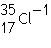has a negative charge, therefore it is an anion.

23.

Elements arranged in horizontal rows in the periodic table are called periods.

Multiple Choice

24.

The particles of matter that have about the same mass as protons  ____.
 a. atoms b. positrons c. isotopes d. neutrons

25.

In which of the following is the number of neutrons correctly represented?
 a.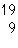F has 28 neutrons. c.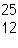Mg has 13 neutrons. b.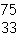As has 108 neutrons. d.U has 330 neutrons.

26.

What unit is used to measure weighted average atomic mass?
 a. amu b. kilogram c. angstrom d. nanogram

27.

Two isotopes of carbon areand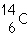If E is the symbol for an element, which two of the following symbols represent isotopes of element E?
1.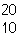E                  2.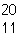E             3.E          4.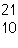E
 a. 1 and 2 b. 3 and 4 c. 1 and 4 d. 2 and 3

28.

Each energy level of an atom has a maximum number of ____ it can hold.
 a. sneutrons b. electron c. quarks d. protons

29.

In a neutral atom the number of protons are equal to the number of ___.
 a. negatrons b. neutrons c. ions d. electrons

30.

Rutherford's experiments led him to conclude that atoms contain massive central regions that have
 a. a positive charge. c. no charge. b. a negative charge. d. both protons and electrons.

31.

An atom is electrically neutral because
 a. neutrons balance the protons and electrons. b. nuclear forces stabilize the charges. c. the numbers of protons and electrons are equal. d. the numbers of protons and neutrons are equal.

32.

Isotopes are atoms of the same element that have different
 a. principal chemical properties. c. numbers of protons. b. masses. d. numbers of electrons.

33.

The tritium atom (Hydrogen-3) consists of
 a. one proton, two neutrons, and two electrons. b. one proton, one neutron, and one electron. c. one proton, two neutrons, and one electron. d. two protons, one neutron, and one electron.

34.

All isotopes of hydrogen contain
 a. one neutron. c. one proton. b. two electrons. d. two nuclei.

35.

Carbon-14 (atomic number 6), the radioactive nuclide used in dating fossils, has
 a. 6 neutrons. c. 10 neutrons. b. 8 neutrons. d. 14 neutrons.

36.

The number of atoms in 1 mol of carbon is
 a. 12 b. 6.02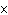1023. c. 6 d. 1

37.

The atomic number of neon is 10. The atomic number of calcium is 20. Compared with a mole of neon, a mole of calcium contains
 a. twice as many atoms. c. an equal number of atoms. b. half as many atoms. d. 20 times as many atoms.

38.

To determine the molar mass of an element, one must know the element's
 a. Avogadro constant. c. number of isotopes. b. atomic number. d. average atomic mass.

39.

Molar mass
 a. is the mass in grams of one mole of a substance. b. is numerically equal to the average atomic mass of the element. c. Both (a) and (b) d. Neither (a) nor (b)

40.

The mass of 2.0 mol of oxygen atoms (atomic mass 16.00 amu) is
 a. 16 g. b. 32 g. c. 48 g. d. 64 g.

41.

A prospector finds 39.39 g of pure gold (atomic mass 196.9665 amu). She has
 a. 1.21023 atoms of Au. c. 4.31023 atoms of Au. b. 2.31023 atoms of Au. d. 6.021023 atoms of Au.

42.

A sample of tin (atomic mass 118.71 amu) contains 3.01 ´ 1023 atoms. The mass of the sample is
 a. 3.01 g. b. 59.3 g. c. 72.6 g. d. 11 g.

43.

If the atomic mass of carbon is 12 u, 1 mole of pure carbon will have a mass of
 a. 6 g. b. 6 mol. c. 12 g. d. 12 mol.

44.

Thomson is responsible for discovering that an atom contains
 a. electrons. b. molecules. c. anodes. d. a nucleus.

45.

What is the mass of 2.5 mol of Ca, which has a molar mass of 40 g/mol?
 a. 2.5 g Ca b. 20.0 g Ca c. 40.0 g Ca d. 100.0 g Ca

46.

A sodium atom, which has 11 electrons, has _____ electron(s) in its third energy level.
 a. 0 b. 1 c. 2 d. 8

47.

Avogadro’s number is defined as the number of particles in
 a. one mole of a substance. c. one gram of a substance. b. one liter of a substance. d. one kilogram of a substance.

48.

Molar mass is defined as
 a. the number of particles in one mole of a substance. b. the SI base unit that describes the amount of a substance. c. the amount of a substance necessary to have a positive charge. d. the mass in grams of one mole of a substance.

49.

The person whose work led to a periodic table based on increasing atomic number was
 a. Moseley. b. Mendeleev. c. Rutherford. d. Cannizzaro.

50.

Which of the following elements is an alkali metal?
 a. calcium-Ca b. magnesium-Mg c. mercury-Hg d. sodium-Na

51.

Most elements are
 a. metals. c. metalloids. b. nonmetals. d. semiconductors.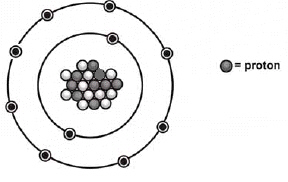52.

What is the mass number of the atom shown above?
 a. 11 b. 18 c. 19 d. 20

53.

Which is the correct symbol for this atom shown above?
 a.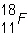1+ b.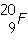1- c.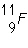d.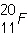1-

54.

All atoms of the same element have the same ____.
 a. number of neutrons c. mass numbers b. number of protons d. mass

55.

Which of the following sets of symbols represents isotopes of the same element?
 a.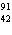J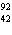JJ c.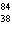M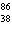M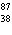M b.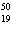L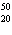L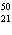L d.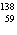QQQ